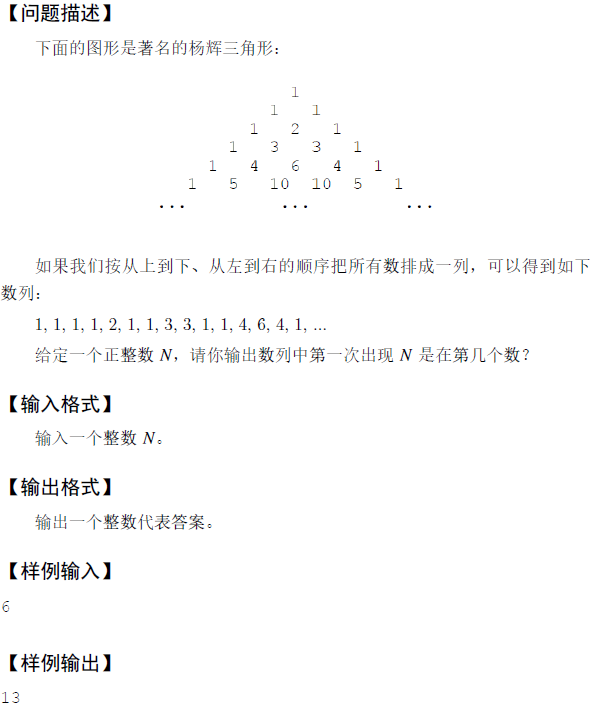# 蓝桥杯—杨辉三角形（C语言解法）

## 题目描述## 代码

``````#include<stdio.h>
int main(){
int n,flag=1;
int num={0};//用来存杨辉三角形的各项
num=1;//为前三项赋值
num=1;
num=1;
int i=2,j=0,count=4;
scanf("%d",&n);
if(n==1)printf("%d",1);//输入为1，直接返回1就行
else{
for(;i<100000000 && flag;i++){
for(j=0;j<=i && flag;j++,count++){
if(j==0){//第一列时特殊处理
num[i][j]=num[i-1][j];
}
num[i][j]=num[i-1][j-1]+num[i-1][j];//下面的数为肩上两数的和
if(num[i][j]==n)flag=0;//找到后退出循环
}
}
printf("%d",count-1);
}
return 0;
}
``````

## 写在最后：不知道为什么总是显示运行错误，想改完全没有头绪啊

### 推荐这些文章：

5
7
3 8
8 1 0
2 7 4 4
4 5 2 6 5

27...

5650 4542 3554 473 946 4114 3871 9073 90 4329
2758 7949 6113 5659 5245 7432 3051 4434 6704 3594
9937 1173 6866 3397 4759 7557 3070 2287 1453 9899
1486 5722 3135 1170 4014 5510 5120 729 2880 9019
2049 698 4582...

#include<stdio.h>
#include<math.h>
int n;
int res;//符合条件的结果数
int st;
int check(int r,int c){
int i;
int t1,t2,t3,t4;
for(i=1;i<...

5
zhangfeng
wangximing
jiujingfazi
woaibeijingtiananmen
haohaoxuexi

772243
344836
297332
716652
875843

#include<stdio.h>
char ch;
int main(){
int n,i,len,sum;
scanf("%d",&n);
while(n--){
sum=0;
scanf("%c",&ch[...

2020 年春节期间，有一个特殊的日期引起了大家的注意：2020 年 2 月 2 日。因为如果将这个日期按 “yyyymmdd” 的格式写成一个 8 位数是 20200202，恰好是一个回文数。我们称这样的日期是回文日期。

2<=N<=100000,0<=Ai<=10^9

5
2 6 4 10 20

10

#include...

1 1
1 2 3

4

#include<stdio.h>
int nm;
int main(){
int i,sum=1,he=0;
nm=1;
for(i=2;i<=20;i++){
sum*=2;
nm[i]=sum;
}
for(i=1;i<=20;i++){
he+=nm/n...

THE END